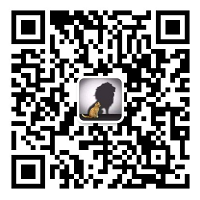# 【飞控】聊点姿态 (二)-坐标系旋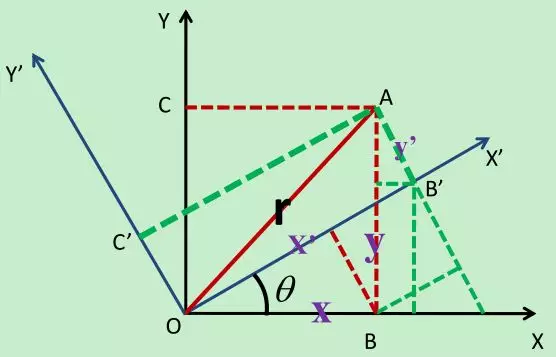$$\begin{matrix} x'=x\cos\theta+y\sin\theta\\ y'=-x\sin\theta+y\cos\theta\\ \end{matrix}$$

$$\left[\begin{matrix} x' \\ y' \end{matrix}\right]=\left[\begin{matrix} \cos\theta & \sin\theta \\ -\sin\theta & \cos\theta \\ \end{matrix}\right]\left[\begin{matrix} x \\ y \end{matrix}\right]$$

$$\left[\begin{matrix} A' \\ B' \end{matrix}\right]=\left[\begin{matrix} \color{blue}\cos\theta & \color{blue}\sin\theta \\ \color{blue}{-\sin}\theta & \color{blue}\cos\theta \\ \end{matrix}\right]\left[\begin{matrix} A \\ B \end{matrix}\right]（√）$$

$$\left[\begin{matrix} x' \\ y' \\z' \end{matrix}\right]=\left[\begin{matrix} \color{blue}\cos\theta & \color{blue}\sin\theta & 0 \\ \color{blue}{-\sin}\theta &\color{blue}\cos\theta & 0 \\ 0 & 0 & 1 \\ \end{matrix}\right]\left[\begin{matrix} x \\ y \\z \end{matrix}\right]（√）$$

$$\left[\begin{matrix} x' \\ y' \\z' \end{matrix}\right]=\left[\begin{matrix} 1 & 0 & 0 \\ 0 &\color{blue}\cos\theta & \color{blue}\sin\theta \\ 0 &\color{blue}{-\sin}\theta & \color{blue}\cos\theta \\ \end{matrix}\right]\left[\begin{matrix} x \\ y \\z \end{matrix}\right]（√）$$

$$\left[\begin{matrix} x' \\ y' \\z' \end{matrix}\right]=\left[\begin{matrix} \color{red}\cos\theta & 0 & \color{red}\sin\theta \\ 0 & 1 & 0 \\ \color{red}{-\sin}\theta & 0 & \color{red}\cos\theta \\ \end{matrix}\right]\left[\begin{matrix} x \\ y \\z \end{matrix}\right] (×)$$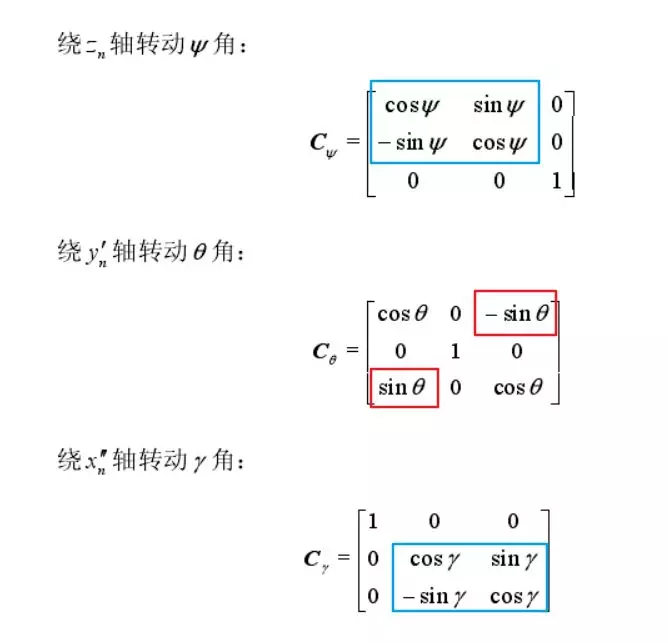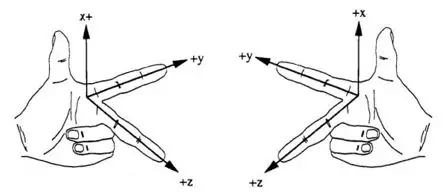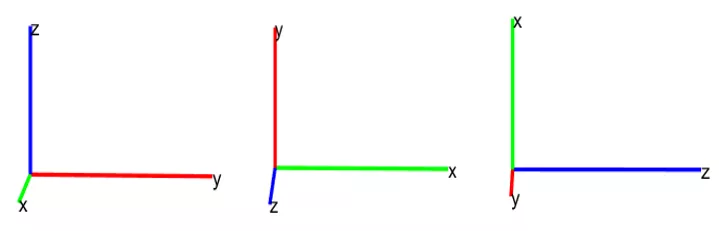$$\begin{matrix} z'=z\cos\theta+x\sin\theta\\ y'=y\\ x'=-z\sin\theta+x\cos\theta\\ \end{matrix}$$

$$\left[\begin{matrix} x' \\ y' \\z' \end{matrix}\right]=\left[\begin{matrix} \color{blue}\cos\theta & 0 & \color{blue}{-\sin}\theta \\ 0 & 1 & 0 \\ \color{blue}\sin\theta & 0 & \color{blue}\cos\theta \\ \end{matrix}\right]\left[\begin{matrix} x \\ y \\z \end{matrix}\right]（√）$$